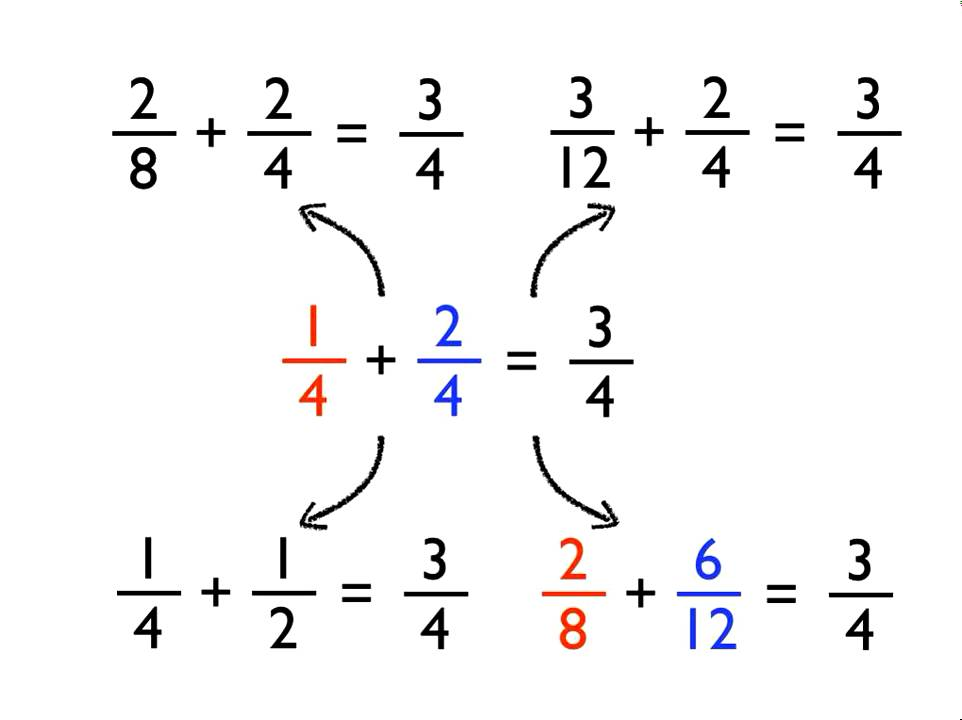Why do students have difficulty in question related to fract - Study24x7New to Study24X7 ?

# Why do students have difficulty in question related to fractions and what can be done to solve the difficulty?

Updated on 17 April 2020Abhishek Kumar
Updated on 17 April 2020Many children never master fractions. When asked whether 12/13 + 7/8 was closest to 1, 2, 19, or 21, only 24% of a nationally representative sample of more than 20,000 US 8th graders answered correctly. This test was given almost 40 years ago. Such difficulties are not limited to fraction estimation problems nor do they end in 8th grade. On standard fraction addition, subtraction, multiplication, and division problems with equal denominators (e.g., 3/5+4/5) and unequal denominators (e.g., 3/5+2/3), 6th and 8th graders tend to answer correctly only about 50% of items. Studies of community college students have revealed similarly poor fraction arithmetic performance. Children in the US do much worse on such problems than their peers in European countries, such as Belgium and Germany, and in Asian countries such as China and Korea.Why are fractions so difficult to understand? A major reason is that learning fractions requires overcoming two types of difficulty: inherent and culturally contingent. Inherent sources of difficulty are those that derive from the nature of fractions, ones that confront all learners in all places.

First inherent difficulty is the notation used to express fractions. Understanding the relation a/b is more difficult than understanding the simple quantity a, regardless of the culture or time period in which a child lives.

Second inherent difficulty involves the complex relations between fraction arithmetic and whole number arithmetic. For example, multiplying fractions involves applying the whole number operation independently to the numerator and the denominator (e.g., 3/7 * 2/7 = (3*2)/(7*7) = 6/49), but doing the same leads to wrong answers on fraction addition (e.g., 3/7 + 2/7 ≠ 5/14).

A third inherent difficulty is complex conceptual relations among different fraction arithmetic operations, at least using standard algorithms. Why do we need equal denominators to add and subtract fractions but not to multiply and divide them? Why do we invert and multiply to solve fraction division problems, and why do we invert the fraction in the denominator rather than the one in the numerator? These inherent sources of difficulty make understanding fraction arithmetic challenging for all students.

A 12-week program designed by Lynn Fuchs (an educational psychologist, who featured in Forbes Magazinein 2009 as one of the 14 "revolutionary educators" nationwide) to help children from low-income backgrounds improve their fraction knowledge, seems especially promising. The program teaches children about fraction magnitudes through tasks such as comparing and ordering fraction magnitudes and locating fractions on number lines. After participating in Fraction Face-off!, fourth graders’ fraction addition and subtraction accuracy consistently exceeds of children receiving the standard classroom curriculum. This finding was especially striking because Fraction Face-off! devoted less time to explicit instruction in fraction arithmetic procedures than did the standard curriculum. Similarly encouraging findings have been found for other interventions that emphasize the importance of fraction magnitudes. Such programs may help children learn fraction arithmetic by encouraging them to note that answers such as 1/3+1/2 = 2/5 cannot be right, because the sum is less than one of the numbers being added, and therefore to try procedures that generate more plausible answers.

Write a comment...Trending ArticlesBy CBSE CLASS 8By CBSE CLASS 10By Examination GuideBy CBSE CLASS 9